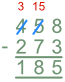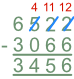Subtracting In Columns

# Subtracting In Columns

Basic, GCSE(F),

Subtraction reduces the value of one number by the value of another. The order of the numbers in the subtraction is important.

Write the two numbers in columns, with the original number on top and the number to be taken away on the bottom.  Line the digits up so that the units are in one column, tens in the next, hundreds in the next column, and so on.

In the units column, check if the bottom number can be subtracted from the top number. If it can, perform the subtraction. If not, take 1 from the next (tens) column and and add 10 to the top number in the units column. The subtraction can now be carried out.

Repeat this process for each column, working right to left.

## Examples

1. Subtract 273 from 458.

Place the original number on the top line, and the number being subtracted on the bottom line. Start the subtraction with the units column.

Calculate 8 – 3 = 5. Put the 5 in the units column. The next calculation is 5 – 7. Seven away from five cannot be done. Take 1 from the hundreds column, which changes the 4 hundred to 3 hundred, and change the 5 to a 15 in the tens. The calculation is now possible: calculate 15 – 7 = 8.

The final step is to calculate the hundreds column: 3 – 2 = 1:2. Coffee sales at the coffee shop were 6522 during the week and 3066 over the weekend.  How many more cups of coffee did the shop sell during the week?